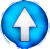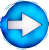Additio or subtrctio of terms - Multiplication of factorsGo ! $2+3\cdot 4$      Here 3 and 4 are factors, 2 and 3 · 4 are terms $2\cdot a - b^2$